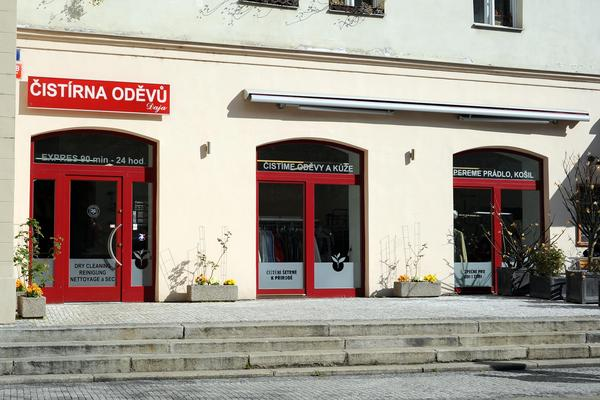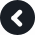Accept

# Dry Cleaning Prague 1

Address: V Celnici 3, Praha 1

Telephone: +420 224 212 787

Opening hours:
Mon - Fri 8.00 am – 8.00 pm, Sat - Sun 8.00 am – 4.00 pm

How to get to us:
Metro station: B - Nám. Republiky
Tram: 3, 6, 8, 14, 15, 24, 26
Parking available on sitex x x x x x x x x x x x x x x x x x x x x x x x x x x x x x x x x x x x x x x x x x x x x x x x x x x x x x x x x x x x x x x x x x x x x x x x x x x x x x x x x x x x x x x x

## Our Awards